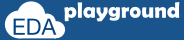# Array reduction method XOR

On calling xor() method, logical xor (^) will be performed on all the array elements and returned.
Let's consider an example of an array with 2, 3 and 4 elements.

Before looking into examples, see to the Truth table for OR.

 A B Y = A ^ B 0 0 0 0 1 1 1 0 1 1 1 0

1. Array with 2 elements.
Consider A=2 and B=3.
Y = A ^ B;
 Binary Decimal A 0010 2 B 0011 3 Y = A ^ B 0001 1

Consider A=2 and B=1.
Y = A ^ B;
 Binary Decimal A 0010 2 B 0001 1 Y = A ^ B 0011 3

2. Array with 3 elements.
Consider A=10, B=9, and C=8.

OR operation of 3 elements performed in 2 steps, In the first step A ^ B will be performed. In the second step result of the first step ^ C will be done. Considering X as the first step result.

X = A ^ B;
Y = X ^ C;
 Binary Decimal A 1010 10 B 1001 9 X = A ^ B 0011 3 C 1000 8 Y = X ^ C 1011 11

2. Array with 4 elements.
Consider A=3, B=5, C=7, and D=9.

Consider X and Y as intermediate results.
X = A ^ B;
Y = X ^ C;
Z = Y ^ D;
 Binary Decimal A 0011 3 B 0101 5 X = A ^ B 0110 6 C 0111 7 Y = X ^ C 0001 1 D 1001 9 Z = Y ^ D 1000 8

Complete code with above examples.

```module fixedsize_array;

//declaration of array’s
int array_1;
int array_2;
int array_3;
int array_4;

int l_xor;

initial begin
//array initialization
array_1  = '{2,3};
array_2  = '{2,1};
array_3  = '{10,9,8};
array_4  = '{3,5,7,9};

l_xor = array_1.xor();
\$display("Logical XOR of array_1 is \t%0d",l_xor);

l_xor = array_2.xor();
\$display("Logical XOR of array_2 is \t%0d",l_xor);

l_xor = array_3.xor();
\$display("Logical XOR of array_3 is \t%0d",l_xor);

l_xor = array_4.xor();
\$display("Logical XOR of array_4 is \t%0d",l_xor);
end

endmodule
```

Simulator Output

Logical XOR of array_1 is 1
Logical XOR of array_2 is 3
Logical XOR of array_3 is 11
Logical XOR of array_4 is 8

## Array reduction methods SUM, PRODUCT using 'with' clause

Condition or expression specified within the with clause will be applied to all the array elements during array reduction methods.

```array_1  = '{1,2,3,4};
array_1.sum with (item * 2);
```

the item indicates the array element.
each array element will be multiplied by 2 and then the sum method will be performed.

Intermediate array elements after multiplication with 2 is  '{2,4,6,12};
sum method will be performed on new array elements. sum = 2+4+6+12;

Below is an example of sum and product methods using with clause.

```module fixedsize_array;

//declaration of array’s
int array_1;

int t_sum,t_product;

initial begin
//array initialization
array_1  = '{1,2,3,4};

t_sum = array_1.sum with (item * 2);
//t_sum = (1*2)+(2*2)+(3*2)+(4*2) = 2+4+6+8
\$display("Sum of array_1 is \t%0d",t_sum);

t_product = array_1.product with (item + 2);
//t_product = (1+2)*(2+2)*(3+2)*(4+2) = 3*4*5*6
\$display("product of array_1 is \t%0d",t_product);
end

endmodule
```

Simulator Output

Sum of array_1 is 20
product of array_1 is 360

Execute the above code on## Array reduction methods AND, OR and XOR using 'with' clause

The operation of these methods is the same as the above example.

```module fixedsize_array;

//declaration of array’s
int array_1;
int array_2;
int array_3;

int b_and,b_or,l_xor;

initial begin
//array initialization
array_1  = '{2,3};
array_2  = '{2,1};
array_3  = '{1,3};

b_and = array_1.and with (item * 2);
\$display("Bit-wise AND of array_1 is \t%0d",b_and);

b_or = array_2.or with (item + 3);
\$display("Bit-wise OR of array_2 is \t%0d",b_or);

l_xor = array_3.xor with (item * 3);
\$display("Logical XOR of array_3 is \t%0d",l_xor);
end

endmodule
```

Simulator Output

Bit-wise AND of array_1 is 4
Bit-wise OR of array_2 is 5
Logical XOR of array_3 is 10

## Array reduction methods on Dynamic and Associative arrays

Examples seen before are on fixed size array, below example is on a dynamic and associative array.

```module dyn_asoc_array;

//declaration of array’s
int dyn_array[];
int asoc_array[*];

int t_sum,t_product;

initial begin
dyn_array = new;

//array initialization
dyn_array  = '{1,2,3,4};

asoc_array = 8;
asoc_array = 2;
asoc_array = 6;
asoc_array = 1;

t_sum = dyn_array.sum(); //t_sum = 1+2+3+4
\$display("Sum of dyn_array is \t\t%0d",t_sum);

t_product = dyn_array.product(); //t_product = 1*2*3*4
\$display("product of dyn_array is \t%0d",t_product);

t_sum = asoc_array.sum(); //t_sum = 8+2+6+1
\$display("Sum of asoc_array is \t\t%0d",t_sum);

t_product = asoc_array.product(); //t_product = 8*2*6*1
\$display("product of asoc_array is \t%0d",t_product);

end

endmodule
```

Simulator Output

Sum of dyn_array is 10
product of dyn_array is 24
Sum of asoc_array is 17
product of asoc_array is 96